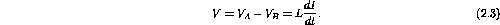Next: Circuit Equations Up: AC Circuit Elements Previous: Capacitance

## Inductance

Faraday's law applied to an inductor states that a changing current induces a back EMF that opposes the change. OrWhere V is the voltage across the inductor and L is the inductance measured in henry (H). The more common units encountered in circuits areH and mH.

The inductance will tend to smooth sudden changes in current just as the capacitance smoothes sudden changes in voltage. Of course, if the current is constant there will be no induced EMF. So unlike the capacitor which behaves like an open-circuit in DC circuits, an inductor behaves like a short-circuit in DC circuits.

Applications using inductors are less common than those using capacitors, but inductors are very common in high frequency circuits. We will again skip over the unpleasantness - that non-ideal inductors have some resistance and some capacitance.

Inductors are never pure inductances because there is always some resistance in and some capacitance between the coil windings. When choosing an inductor (occasionally called a choke) for a specific application, it is necessary to consider the value of the inductance, the DC resistance of the coil, the current-carrying capacity of the coil windings, the breakdown voltage between the coil and the frame, and the frequency range in which the coil is designed to operate. To obtain a very high inductance it is necessary to have a coil of many turns. The inductance can be further increased by winding the coil on a closed-loop iron or ferrite core. To obtain as pure an inductance as possible, the DC resistance of the windings should be reduced to a minimum. This can be done by increasing the wire size, which of course, increases the size of the choke. The size of the wire also determines the current-handling capacity of the choke since the work done in forcing a current through a resistance is converted to heat in the resistance. Magnetic losses in an iron core also account for some heating, and this heating restricts any choke to a certain safe operating current. The windings of the coil must be insulated from the frame as well as from each other. Heavier insulation, which necessarily makes the choke more bulky, is used in applications where there will be a high voltage between the frame and the winding. The losses sustained in the iron core increases as the frequency increases. Large inductors, rated in henries, are used principally in power applications. The frequency in these circuits is relatively low, generally 60 Hz or low multiples thereof. In high-frequency circuits, such as those found in FM radios and television sets, very small inductors (of the order of microhenries) are frequently used.Next: Circuit Equations Up: AC Circuit Elements Previous: Capacitance

Doug Gingrich
Tue Jul 13 16:55:15 EDT 1999